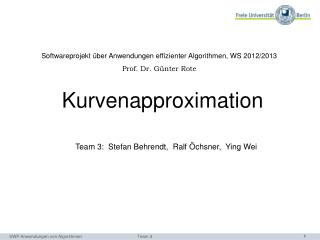DownloadDownload PresentationSoftwareprojekt über Anwendungen effizienter Algorithmen, WS 2012/2013 Prof . Dr . Günter Rote

# Softwareprojekt über Anwendungen effizienter Algorithmen, WS 2012/2013 Prof . Dr . Günter Rote

Télécharger la présentation## Softwareprojekt über Anwendungen effizienter Algorithmen, WS 2012/2013 Prof . Dr . Günter Rote

- - - - - - - - - - - - - - - - - - - - - - - - - - - E N D - - - - - - - - - - - - - - - - - - - - - - - - - - -
##### Presentation Transcript

1. Softwareprojekt über Anwendungen effizienter Algorithmen, WS 2012/2013 Prof. Dr. Günter Rote • Kurvenapproximation Team 3: Stefan Behrendt, Ralf Öchsner, Ying Wei SWP Anwendungen von Algorithmen Team 3

2. Outline • Our task • Technique • Technologies • Flow of process • Preparations for Greedy approach • Greedy approach • Analysis • Demo …. SWP Anwendungen von Algorithmen Team 3

3. Our task • Approximation of point sequences by spline • Input : The points with an order • A predefined tolerance error • Output : A Curve, which goes through the selected points from original given points and can sufficiently good approximate the original point sequence SWP Anwendungen von Algorithmen Team 3

4. Technique • Technologies : • C++, Lua, IPE • QT • Cubic Hermite spline, Cubic Bezier spline, Interpolation • Greedy Algorithm SWP Anwendungen von Algorithmen Team 3

5. Technique • Flow of process 1) Convert given points and computed Tangents to Bezier control points in order to prepare with Greedy 2) Select points & Tangents using Greedy 1) Convert Selected Hermite Points & Tangents to Bezier control points 2) Then draw curve again by Bezier cubic spline Calculate all Tangents of original given Points Add spline into IPE for display SWP Anwendungen von Algorithmen Team 3

6. Tangents Calculation • i is index of given points (points[i]), n is the size of given points • for(i=0; i <n; i++) • if (i==0) then • else if (i==n-1) then • else • end if • T(n)=n SWP Anwendungen von Algorithmen Team 3

7. Preparations for Greedy Approach • n=size of given points; T(n)=n • is defined as maximal error from beginning to the current position : • m=size of cPoints; T(n)=n • , , is defined as minimal distance between points[i] and cubic Bezier interpolating points: • , where • : cubic Bezier Curve. T(n)=1 • Let , interpolating could be realized. SWP Anwendungen von Algorithmen Team 3

8. Greedy Approach • 1). Add points and its tangent into hermitePoint and tangents • 2). for (i=1 to (size of given points -1), i++) • 2.1) Add points[i] and its tangent into hermitePoint[i] and tangents[i] • 2.2) Convert hermite points and tangents to cubic Bezier control points • i=0; • for(j=1 to (size of hermitePoints -1), j++) • i++; SWP Anwendungen von Algorithmen Team 3

9. Greedy Approach • 2.3) select points and tangents • 2.3.1) • if the error in the position i ≤ tolerance error ε , and i isn’t end position • ( errorUntil(i) < maxError && i != (size of points -1) ) • then a.) Adjust the length of tangent • , where • b.) Remove this point from hermitePoints[end]; • Remove its tangents from tangents[end]; SWP Anwendungen von Algorithmen Team 3

10. Greedy Approach 2.3.2) else if at the end, and the error >maxError (i==(size of points-1) && (errorUntil(i)>Error)) then insert points[i-1] into hermite[end-1]; insert tangent[i-1] into tangents[end-1]; 2.3.3) else (when the error > maxError), insert points[i-1] into hermitePoints[end-1]; insert tangent[i-1] into tangents[end-1]; 3) Convert the selected hermitePoints to cPoints again. Method is the same with 2.2) T(n)=n 4) add bezier spline to IPE SWP Anwendungen von Algorithmen Team 3

11. Analysis • Runtime: • n is the size of inputs • Tangents Calculation : O(n) • Greedy approach : O(n*n) • Convert selected hermitePoints to cPoints : O(n) • Memory requirements := • In worse case, memory requirements := SWP Anwendungen von Algorithmen Team 3

12. Analysis • We chose four example functions: • sine in range [0 ; 10] • square root function in range [0 ; 10] • polynomial function of 4th degree: x⁴ – 2x² in range [-2 ; 2] • polynomial function of 5th degree: x⁵ + 3.5x⁴ – 2.5x³ – 12.5x² +1.5x + 9 in range [-3 ; 1.5] SWP Anwendungen von Algorithmen Team 3

13. Analysis • Evaluating our measurement results, we can formulate some conclusions about the behaviour of the algorithm under several circumstances: • Generally, the compression increases with higher („less strict“) error limits. • Runtime and compression are proportional; that means if we watch the calculations with a point set and different error values, the runtime and compression increase / decrease in the same way • The more adjacent the points are, the longer takes the computation, but the compression becomes smaller (except for the square root function) • Point sets of polynomial functions cannot be approximated with high precision; usually a „real“ compression (below 100%) starts with an error value of 5 to 10 • The sine curve can be best approximated with many given adjacent points; the square root worst • The last point can lead us to the speculation, that point sets of curves with many similar „curve segments“ (like a sine) can be approximated best, whereas curves which cannot really be seperated, have the worst compression results. We have seen that the first polynomial function of 4th degree is better compressible than the polynomial function of 5th degree, which has less „similar“ curve segments. SWP Anwendungen von Algorithmen Team 3

14. Analysis SWP Anwendungen von Algorithmen Team 3

15. Analysis SWP Anwendungen von Algorithmen Team 3

16. Analysis SWP Anwendungen von Algorithmen Team 3

17. Demo DEMO… SWP Anwendungen von Algorithmen Team 3

18. Thanks For Attention! Questions and Suggestions for improvement?  SWP Anwendungen von Algorithmen Team 3